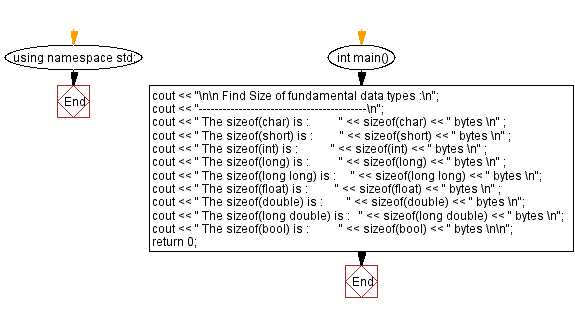﻿ C++ Exercises: Find Size of fundamental data types - w3resource

# C++ Exercises: Find Size of fundamental data types

## C++ Basic: Exercise-3 with Solution

Write a in C++ program to find the size of fundamental data types.

Sample Solution:

C++ Code :

``````#include <iostream>
using namespace std;

int main()
{
cout << "\n\n Find Size of fundamental data types :\n";
cout << "------------------------------------------\n";
cout << " The sizeof(char) is :          " << sizeof(char) << " bytes \n" ;
cout << " The sizeof(short) is :         " << sizeof(short) << " bytes \n" ;
cout << " The sizeof(int) is :           " << sizeof(int) << " bytes \n" ;
cout << " The sizeof(long) is :          " << sizeof(long) << " bytes \n" ;
cout << " The sizeof(long long) is :     " << sizeof(long long) << " bytes \n";
cout << " The sizeof(float) is :         " << sizeof(float) << " bytes \n" ;
cout << " The sizeof(double) is :        " << sizeof(double) << " bytes \n";
cout << " The sizeof(long double) is :   " << sizeof(long double) << " bytes \n";
cout << " The sizeof(bool) is :          " << sizeof(bool) << " bytes \n\n";
return 0;
}
``````

Sample Output:

``` Find Size of fundamental data types :
------------------------------------------
The sizeof(char) is :          1 bytes
The sizeof(short) is :         2 bytes
The sizeof(int) is :           4 bytes
The sizeof(long) is :          8 bytes
The sizeof(long long) is :     8 bytes
The sizeof(float) is :         4 bytes
The sizeof(double) is :        8 bytes
The sizeof(long double) is :   16 bytes
The sizeof(bool) is :          1 bytes
```

Flowchart:C++ Code Editor:

What is the difficulty level of this exercise?

﻿

## C++ Programming: Tips of the Day

Why is there no std::stou?

The most pat answer would be that the C library has no corresponding "strtou", and the C++11 string functions are all just thinly veiled wrappers around the C library functions: The std::sto* functions mirror strto*, and the std::to_string functions use sprintf.

Ref: https://bit.ly/3wtz2qA

We are closing our Disqus commenting system for some maintenanace issues. You may write to us at reach[at]yahoo[dot]com or visit us at Facebook